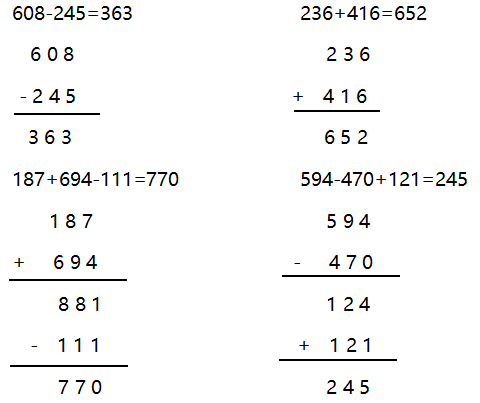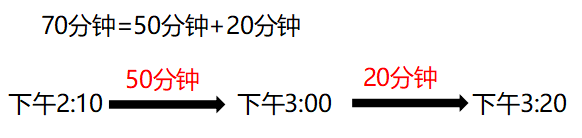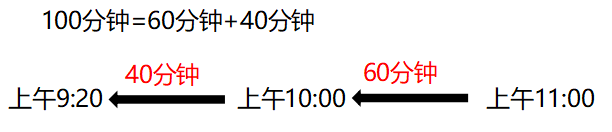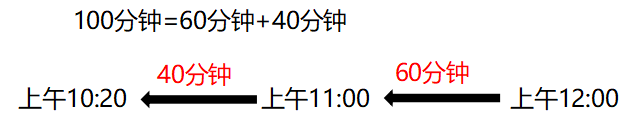# 人教版小学数学三年级上册期中测试卷及答案

1.540是由（ ）个百和（ ）个十组成的。

2.与299相邻的两个数是（ ）和（ ）。

3.估算203+499时，把203看作（ ），把499看作（ ），所以估算的结果是（ ）。

4.两个减数的差是160，若被减数是476，那么减数是（ ）；若减数是362，那么被减数是（ ）。

5.最大的三位数与最小的三位数的差是（ ）。

6.在一个加法算式中，一个加数增加382，另一个加数不变，那么和会（ ）。

7.小明从家走到学校大约需要20（ ）。（填“小时“、“分钟”或”秒”）

200分米=（ ）米 500厘米=（ ）分米

4000米=（ ）千米 2800分米=（ ）米

2千米=（ ）米 1700厘米=（ ）米

3千米-500米=（ ）米 2千米300米=（ ）米

3吨=（ ）千克 5000千克=（ ）吨

1吨203千克=（ ）千克 6吨9千克=（ ）千克

4000克=（ ）千克 8千克90克=（ ）克

5吨-280千克=（ ）千克 9克+2千克=（ ）克

3时=（ ）分 1分39秒=（ ）秒

3时-60分=（ ）时 110分=（ ）时（ ）分

1.小明今天中午12:10开始吃午饭，用了26分钟吃完，那么小明吃完午饭的时间是12:36。 （ ）

2.2吨的铁比2000千克的棉花重。 （ ）

3.从西安到上海的铁路长2878厘米。 （ ）

4.503-199的结果大约是400。 （ ）

5.一本《现代汉语词典》的厚度约为5厘米。 （ ）

1.爸爸的身高约是182（ ）。

A.毫米 B.厘米 C.米

2.千米和米之间的进率是（ ）。

A.1000 B.100 C.10

3.一只大象约重7（ ）。

A.克 B.千克 C.吨

4.499+201-504的结果大约是（ ）。

A.100 B.200 C.300

5.两位数与三位数相加，结果（ ）。

A.一定是三位数

B.一定是四位数

C.可能是四位数

1.口算。（6分）

480-70= 310+150=

490-130= 510-240=

1000-650+20= 320+160-200=

2.列竖式计算。（4分）

608-245= 236+416=

187+694-111= 594-470+121=

3.脱式计算。（6分）

89+111-5×4 9×9+158

591-322÷7 845-（126+245）

81÷9+211 460+300-297

1.妈妈今天要去做瑜伽，瑜伽课是下午2:10分开始，70分钟后结束，那么瑜伽课结束是在什么时间？（5分）

2.阳光小学举办足球比赛，分为上、下两个半场，上半场45分钟，然后中间休息10分钟，再接着进行下半场。已知两支足球队在上午11:00比赛结束。（6分）

（1）整个足球比赛共用多长时间？

（2）这场足球比赛是在什么时间开始的？

（3）若想在上午12:00结束比赛，比赛应该在什么时间开始？

3.一家水果店上周卖出291千克的水果，这周卖出336千克水果，下周再卖出多少千克水果这三周就一共卖出去1吨水果？（5分）

4.修路队修一条路，第一天修了这条路的一半还多300米，第二天修了余下的一半，此时还剩810米没有修。这条路全长多少米？（6分）

1.5,4

2.298,300

3.200，500,700

4.316,522

5.899

6.增加382

7.分钟

200分米=（ 20 ）米 500厘米=（ 50 ）分米

4000米=（ 4 ）千米 2800分米=（ 280 ）米

2千米=（ 2000 ）米 1700厘米=（ 17 ）米

3千米-500米=（ 2500 ）米 2千米300米=（2300）米

3吨=（ 3000 ）千克 5000千克=（ 5 ）吨

1吨203千克=（1203）千克 6吨9千克=（ 6009 ）千克

4000克=（ 4 ）千克 8千克90克=（ 8090 ）克

5吨-280千克=（4720）千克 9克+2千克=（ 2009 ）克

3时=（ 180 ）分 1分39秒=（ 99 ）秒

3时-60分=（ 2 ）时 110分=（ 1 ）时（ 50 ）分

√ × × × √

B A C B C

1.

480-70=410 310+150=460

490-130=360 510-240=270

1000-650+20=370 320+160-200=280

2.3.

89+111-5×4 9×9+158

=200-20 =81+158

=180 =239

591-322÷7 845-（126+245）

=591-46 =845-371

=545 =474

81÷9+211 460+300-297

=9+211 =760-297

=220 =463

1.2.（1）45+10+45=100（分钟）

（2）（3）3.1吨=1000千克 1000-291-336=373（千克）

4.810×2=1620（米） （1620+300）×2=3840（米）

###热门文章推荐95.6%的用户看完本文后，还关注了以下精彩公众号